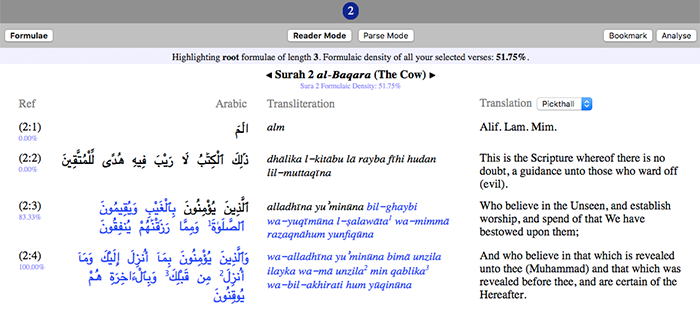Qur’an Tools is not optimised for narrow screens

Underlying the Arabic text of the Qur’an is an extensive network of formulaic diction — short repeated phrases that occur time and time again. Built into Qur’an Tools is a powerful suite of tools to enable you to explore, study, and analyse with these formula.

#### What is a Formula?

A formula is simply a group of words that are repeated more than once in the Qur’an. For example, the formula 'mn + ‘ml + ṣlḥ (i.e. the Arabic root 'mn followed by the root 'ml followed by the root ṣlḥ)appears 61 times in the Qur’an. You can see every formula that occurs in the Qur’an by choosing ‘List All Formulae’ from the ‘Formulae’ menu in the Qur’an Tools menu bar.

#### Formula Types

The qur’anic formulae recorded in Qur’an Tools fall into three types:

• Root Formulae — like 'mn + ‘ml + ṣlḥ above, a root formula is a set of Arabic roots that occur following each other (any particles or pronouns that appear in between are simply ignored).

• Roots plus Particles/Pronouns — in this case, the formula has a “slot” built into it where a particle or a pronoun must appear, in order for the formula’s occurence to be counted in the text. For example, this formula appears 37 times in the Qur’an:

jry + [PART/PRON] + tḥt + nhr

The root jry must be followed by a particle or pronoun, then by the root tḥt, then by the root nhr. This formula occurs in Q. 2:25, where we have:

tajrī [root: jry] min [particle] taḥtihā [root: tḥt] l‑anhāru [root: nhr]

• Lemmata Formula — in this case, rather than Arabic roots, the formula consists of lemma — the inflected dictionary form of the Arabic word. For example, here is a lemmata formula that occurs 50 times in the Qur’an:

alladhī + 'āmana + ʿamila + ṣāliḥāt

#### Formula Length

As well as different types of formulae, there are also different lengths. Qur’an Tools records all formulae are 3, 4 or 5 items long. Here are some examples:

 Type Length: 3 Length: 4 Length: 5 Root 'mn + ‘ml + ṣlḥ jnn + jry + tḥt + nhr 'rḍ + nẓr + kyf + kwn + ‘qb Root plus Particles/Pronouns ‘lm + [PART/PRON] + 'lh jry + [PART/PRON] + tḥt + nhr 'lh + [PART/PRON] + kll + shy' + qdr Lemmata ayyuhā + lladhī + 'āmana 'inn + fī + dhālik + 'āyat fī + samā' + mā + fī + arḍ

#### Working with Formula

Here are a few of the many things you can do with formulae in Qur’an Tools:

1. See the formulaic density of the entire Qur’an (the percentage of the entire qur’anic text that is formulaic) at a glance using the Formulaic Density Summary Table.

2. View a chart of the formulaic density of individual suras of the Qur’an; choose ‘Charts’, then ‘Formulae’, then ‘Formulaic Density per Sura’.

3. Explore a list of all the formulae in the Qur’an by choosing ‘Formulae’ and then ‘List All Formulae’.

4. Easily see all the formulae in any passage in the Qur’an: for example, open sura 2 in the verse browser, then click the Formula button at the top left of the screen, choose the formulae type and length you want, then click OK.5. Quickly cross-reference all the formulae in a passage and see at a glance where else they appear in the Qur’an. For example, open sura 2 in the verse browser, then click the Formula button at the top left of the screen, then click ‘Cross Reference Formulae in Suras’.#### Formulaic Density and Orality

There is a strong argument that a text’s having a high formulaic density, as the Qur’an does, is a very significant indicator that the text (or portions thereof) were originally constructed, live-in-performance, by an oral performer. For more on this thesis, read Dr. Andrew Bannister’s essay,  ‘Retelling the Tale: A Computerised Oral-Formulaic Analysis of the Qur’an’, or his book, An Oral-Formulaic Study of the Qur’an (Lexington, 2017 ).